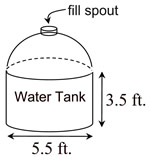Geomery section of MathBitsNotebook.comPractice Test 2 - CCSS Geometry Interactive Version - Long ResponseDirections:  Questions for Parts II, III, and IV are long response questions. You will need paper, pencil and a graphing calculator to work out the problems. Check your answer after solving each problem by clicking the ANSWER button.
Part II: (2 pts)
 25. In circle O, diameter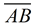has a length of 15 cm. and m∠BAC = 65º. Find the area of sector BOC of the circle. Express the answer to the nearest tenth of a square cm.
 26. ΔABC is translated by the rule: (x, y) → (x - 6, y + 8). a. Is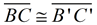? Explain. b. If B(4,-2), what is BB' ?
 27. The triangular prism and the rectangular prism, shown at the right, have been sliced by a plane parallel to their bases creating the shaded cross sections. a. Find the areas of the two cross sections. b. Find the volumes of the two figures.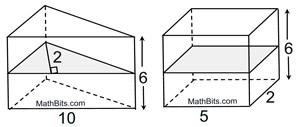28. In square PQRS, diagonal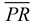has coordinates P(-2,5) and R(6,-1). Write the equation of the line that contains the other diagonal of the square,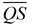.
 29. Given ΔABC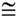ΔEBD, AC = 3x + 4, ED = 5x - 4 and AB = 4x + 5. Find EB.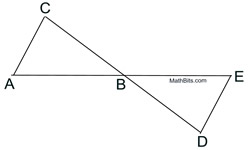30. Triangle A(2,8), B(4,4) and C(8,8) is plotted. A dilation of scale factor ½, centered at point A, is applied. Plot the image ΔA'B'C' and state the coordiantes of its vertices.
 31. Using a compass and straightedge, construct the median to sideof ΔABC.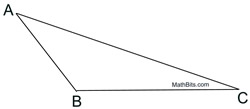Part III:
(4 pts)
 32. Points M(3,0), N(7,0) and P(3,3) are plotted to form ΔPMN. The triangle is rotated about the x-axis. a. What three dimensional figure is formed by the rotation? b. Find the volume of the three dimensional figure to the nearest tenth of a cubic unit.
 33. Parallelogram ABCD is plotted with coordinates A(0, 0), B(2k, 0), C(2k+2n, 2m) and D(2n, 2m). Show that the diagonals bisect each other.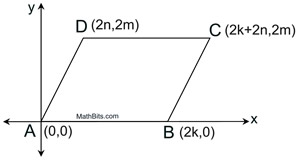34. From point P, on the ground, 45 feet from the foot of an industrial building, the angle of elevation of the bottom of a window, A, is 40º. The angle of eleveation of the top of the window, B, is 50º. Find the height of the window, from A to B, to the nearest foot.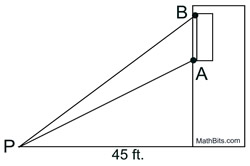Part IV:
(6 pts)
 35.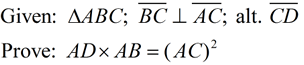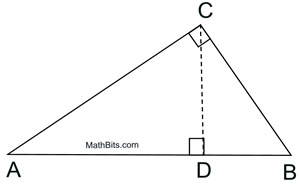36. A new swimming pool is an 18 feet wide and 36 feet long rectangle with varying depths. The diagram at the right shows a cross-section view of the water depth dimensions.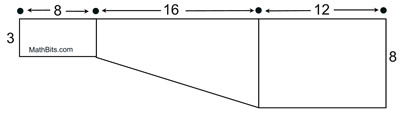a) If 1 cubic foot = 7.48 gallons of water, how mnay gallons of water will be needed to fill the pool, to the nearest gallon? b) To save money, Kyle decides to haul the water for the pool with his pickup truck and a rented water tank that he will place in the back of the truck. The rented water tank has a cylindrical base with a 5.5 foot diameter and a hemispherical (half a sphere) top. The cargo box of Kyle's truck has a carrying capacity of 3000 pounds. Assume that Kyle will fill the tank to its maximum capacity and that water weighs 8.34 pounds per gallon. Will Kyle be able to safely transport the water? Justify your answer.